# RD Sharma Solutions for Class 11 Chapter 17 - Combinations Exercise 17.1

In this section, we shall discuss some important properties of combinations C(n,r). Students who find difficulty in understanding the concepts and solving problems can make use of pdf of solutions. Our expert faculty team at BYJU’S having vast experience in their respective fields have designed the solutions. The problems are solved in a structured manner so that it helps students understand each step, as each step carries marks in the board exam. Students can improve their problem solving and logical thinking abilities by using RD Sharma Class 11 Maths Solutions pdf, from the links given below.

## Download the pdf of RD Sharma Solutions for Class 11 Maths Exercise 17.1 Chapter 17 – Combinations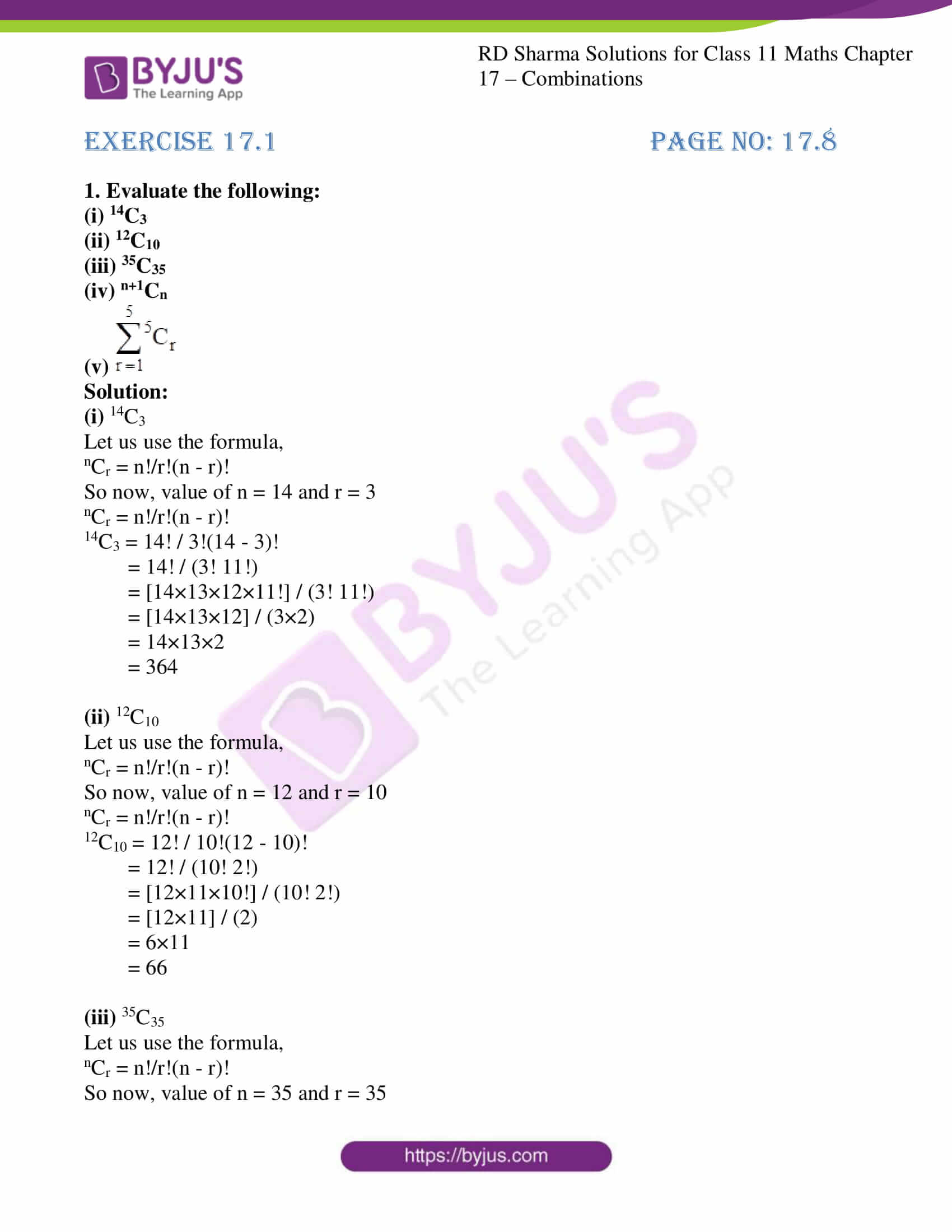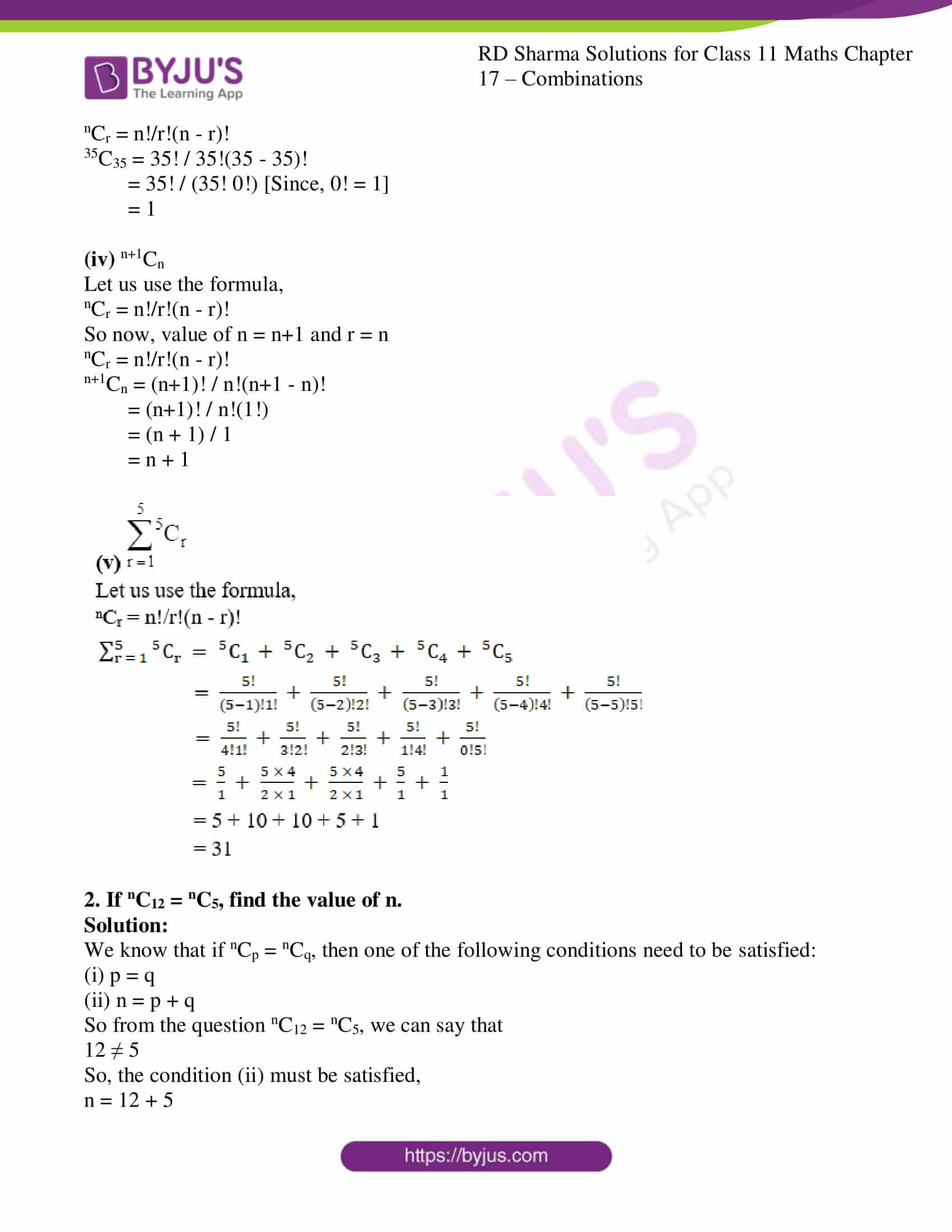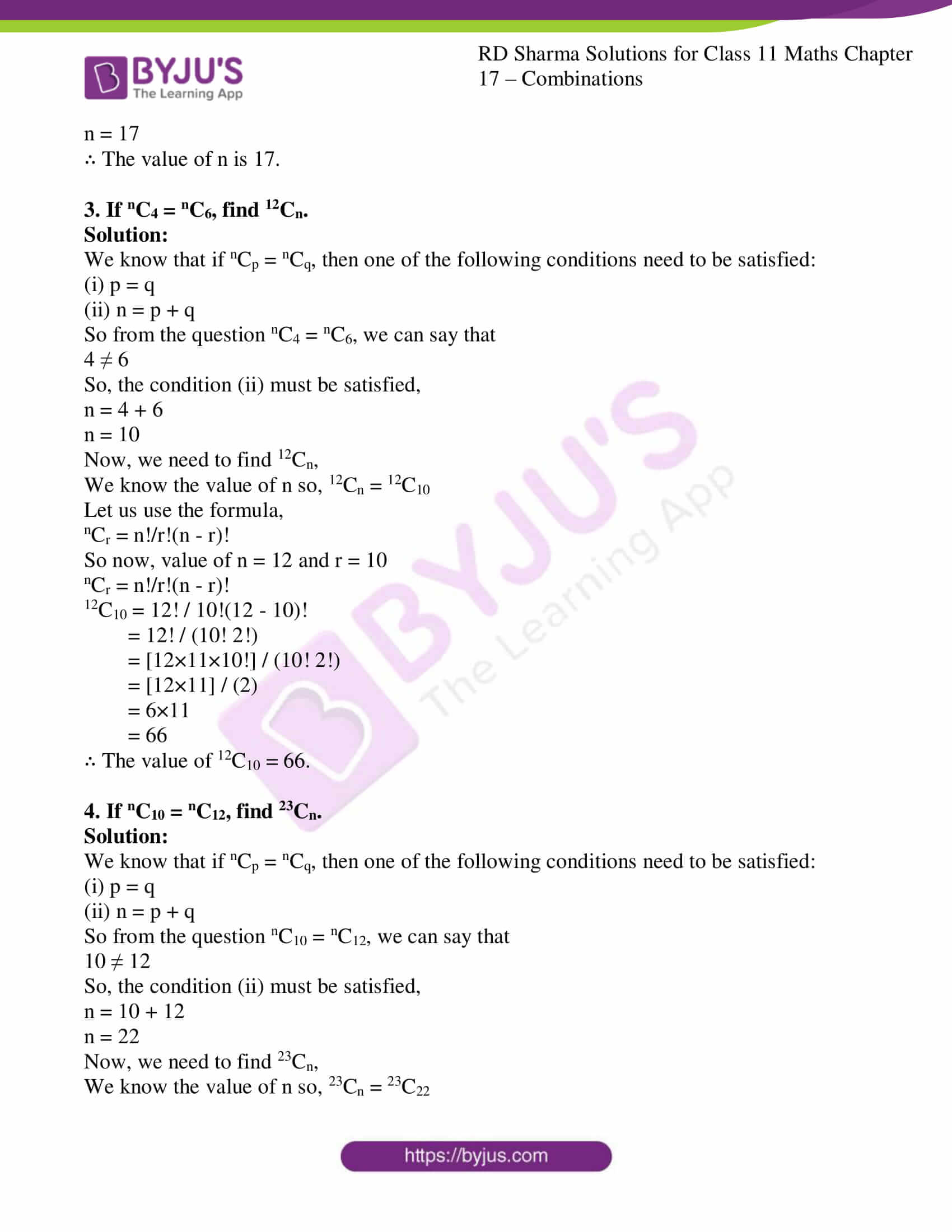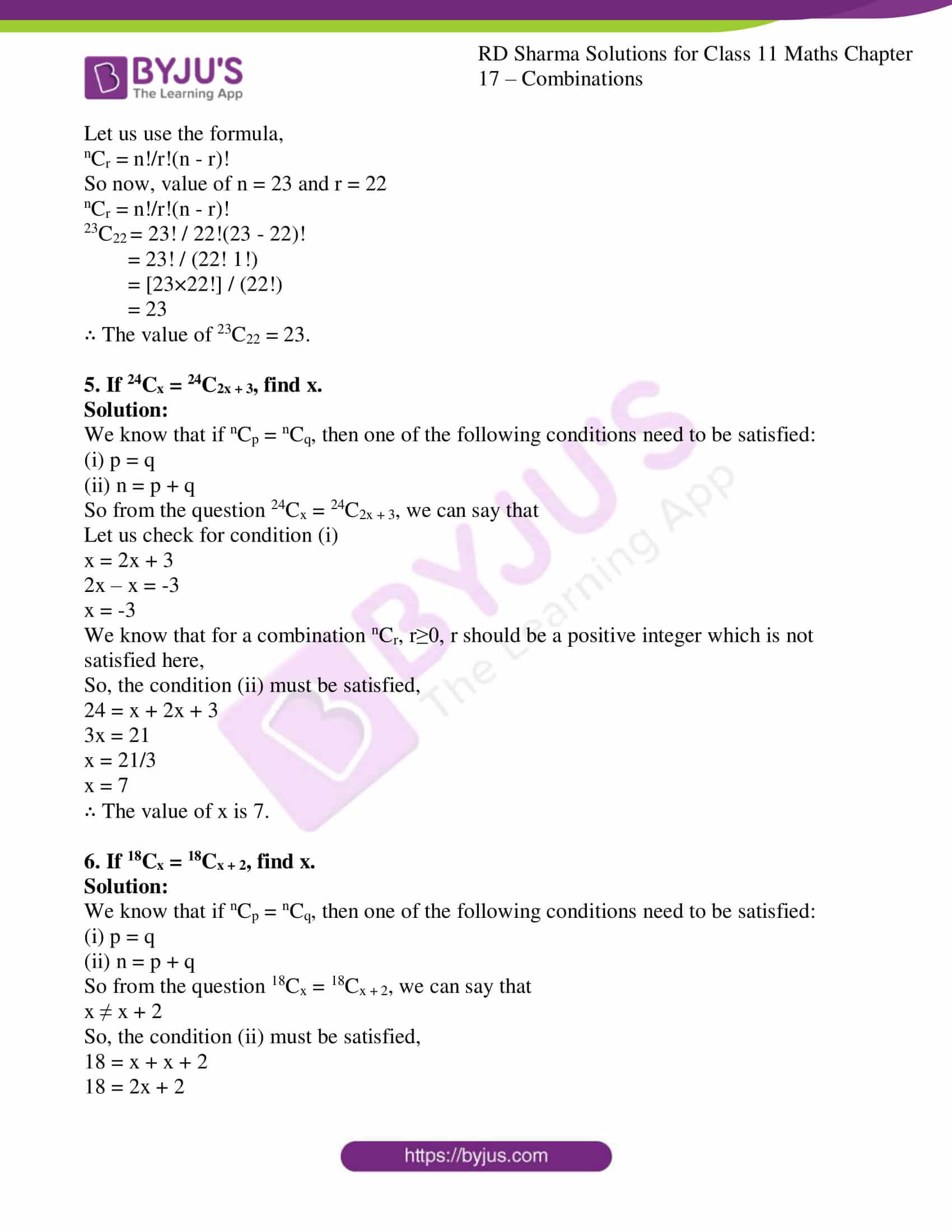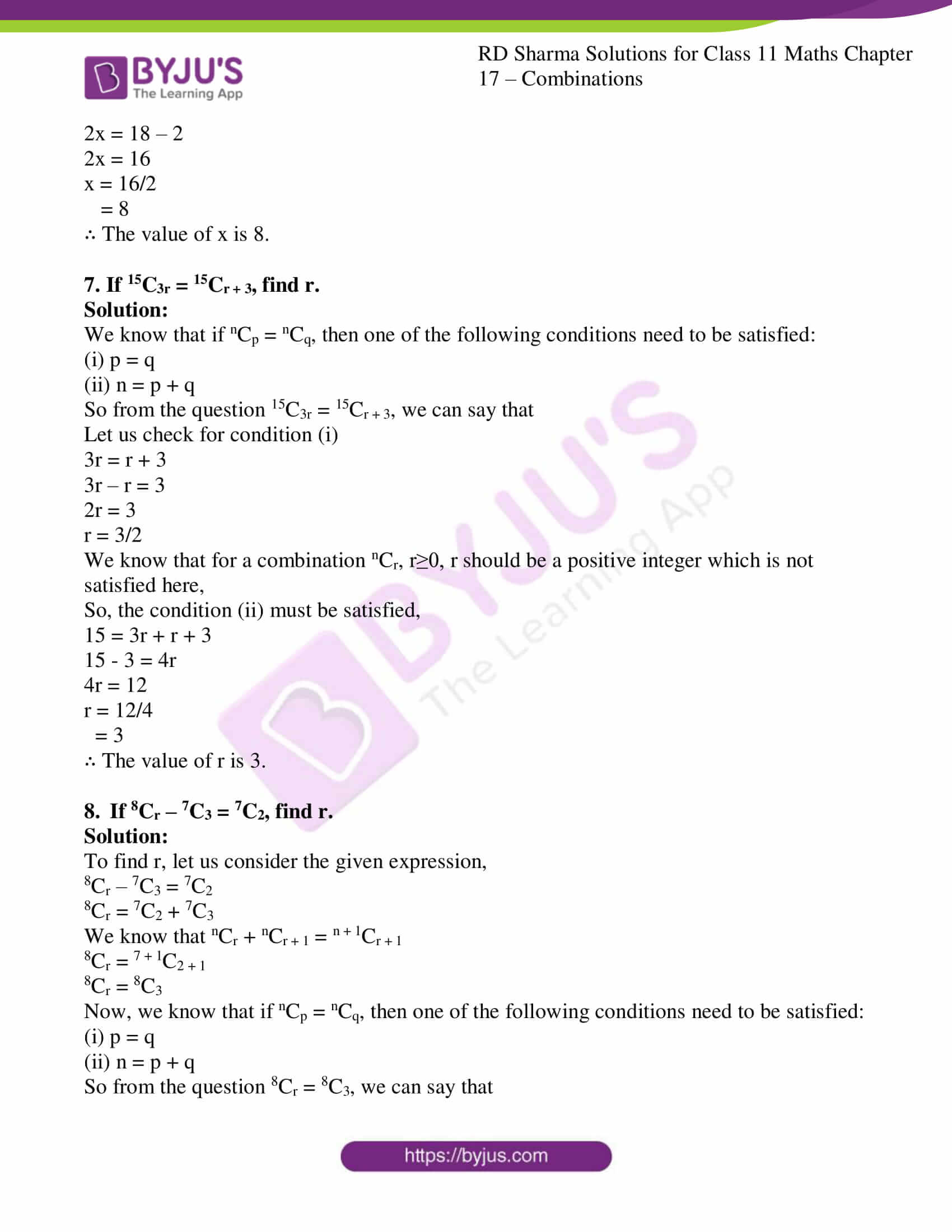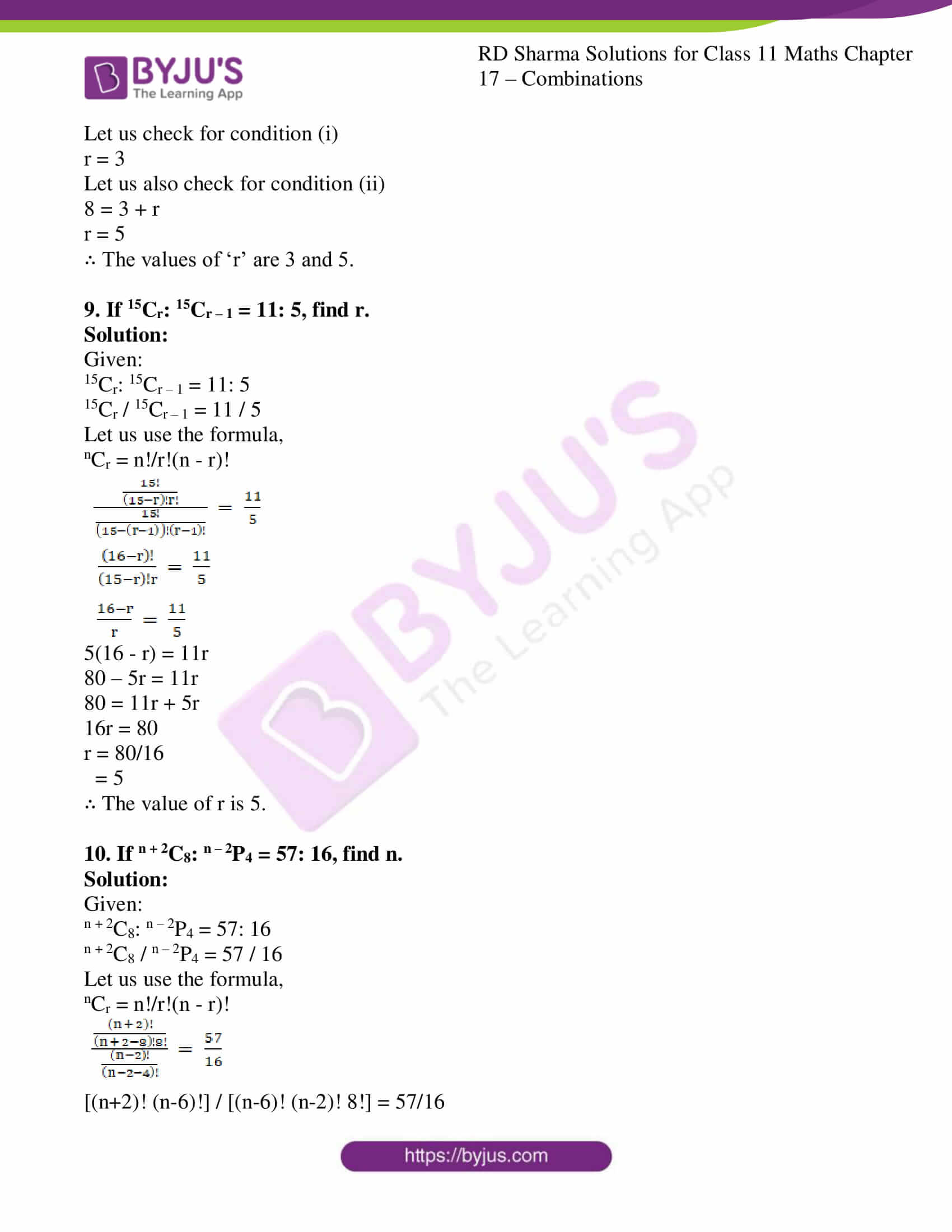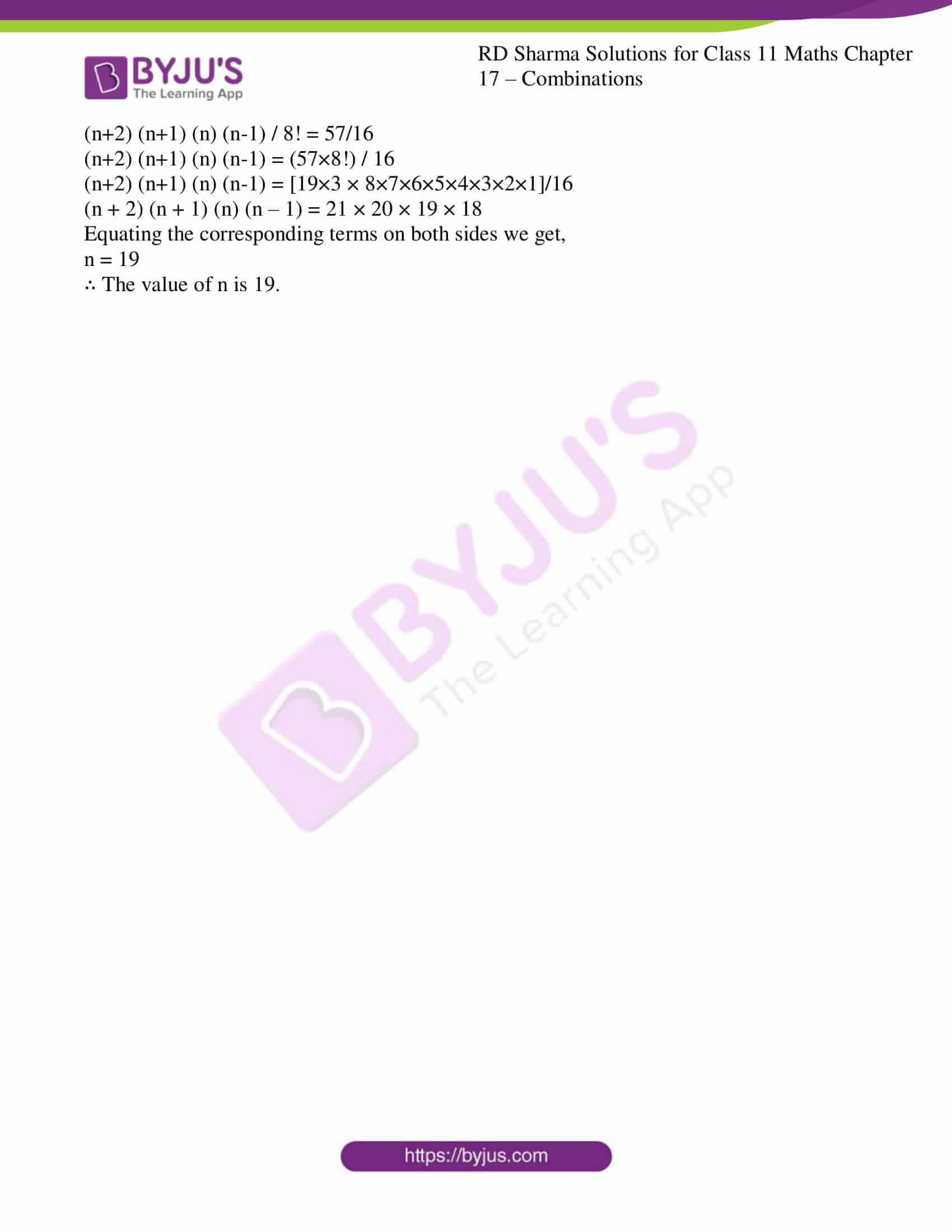### Access answers to RD Sharma Solutions for Class 11 Maths Exercise 17.1 Chapter 17 – Combinations

1. Evaluate the following:

(i) 14C3

(ii) 12C10

(iii) 35C35

(iv) n+1Cn

(v)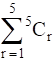Solution:

(i) 14C3

Let us use the formula,

nCr = n!/r!(n – r)!

So now, value of n = 14 and r = 3

nCr = n!/r!(n – r)!

14C3 = 14! / 3!(14 – 3)!

= 14! / (3! 11!)

= [14×13×12×11!] / (3! 11!)

= [14×13×12] / (3×2)

= 14×13×2

= 364

(ii) 12C10

Let us use the formula,

nCr = n!/r!(n – r)!

So now, value of n = 12 and r = 10

nCr = n!/r!(n – r)!

12C10 = 12! / 10!(12 – 10)!

= 12! / (10! 2!)

= [12×11×10!] / (10! 2!)

= [12×11] / (2)

= 6×11

= 66

(iii) 35C35

Let us use the formula,

nCr = n!/r!(n – r)!

So now, value of n = 35 and r = 35

nCr = n!/r!(n – r)!

35C35 = 35! / 35!(35 – 35)!

= 35! / (35! 0!) [Since, 0! = 1]

= 1

(iv) n+1Cn

Let us use the formula,

nCr = n!/r!(n – r)!

So now, value of n = n+1 and r = n

nCr = n!/r!(n – r)!

n+1Cn = (n+1)! / n!(n+1 – n)!

= (n+1)! / n!(1!)

= (n + 1) / 1

= n + 1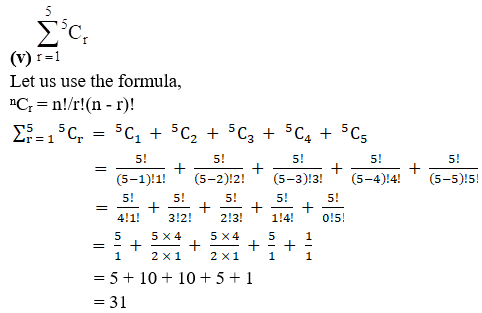2. If nC12 = nC5, find the value of n.

Solution:

We know that if nCp = nCq, then one of the following conditions need to be satisfied:

(i) p = q

(ii) n = p + q

So from the question nC12 = nC5, we can say that

12 ≠ 5

So, the condition (ii) must be satisfied,

n = 12 + 5

n = 17

∴ The value of n is 17.

3. If nC4 = nC6, find 12Cn.

Solution:

We know that if nCp = nCq, then one of the following conditions need to be satisfied:

(i) p = q

(ii) n = p + q

So from the question nC4 = nC6, we can say that

4 ≠ 6

So, the condition (ii) must be satisfied,

n = 4 + 6

n = 10

Now, we need to find 12Cn,

We know the value of n so, 12Cn = 12C10

Let us use the formula,

nCr = n!/r!(n – r)!

So now, value of n = 12 and r = 10

nCr = n!/r!(n – r)!

12C10 = 12! / 10!(12 – 10)!

= 12! / (10! 2!)

= [12×11×10!] / (10! 2!)

= [12×11] / (2)

= 6×11

= 66

∴ The value of 12C10 = 66.

4. If nC10 = nC12, find 23Cn.

Solution:

We know that if nCp = nCq, then one of the following conditions need to be satisfied:

(i) p = q

(ii) n = p + q

So from the question nC10 = nC12, we can say that

10 ≠ 12

So, the condition (ii) must be satisfied,

n = 10 + 12

n = 22

Now, we need to find 23Cn,

We know the value of n so, 23Cn = 23C22

Let us use the formula,

nCr = n!/r!(n – r)!

So now, value of n = 23 and r = 22

nCr = n!/r!(n – r)!

23C22 = 23! / 22!(23 – 22)!

= 23! / (22! 1!)

= [23×22!] / (22!)

= 23

∴ The value of 23C22 = 23.

5. If 24Cx = 24C2x + 3, find x.

Solution:

We know that if nCp = nCq, then one of the following conditions need to be satisfied:

(i) p = q

(ii) n = p + q

So from the question 24Cx = 24C2x + 3, we can say that

Let us check for condition (i)

x = 2x + 3

2x – x = -3

x = -3

We know that for a combination nCr, r≥0, r should be a positive integer which is not satisfied here,

So, the condition (ii) must be satisfied,

24 = x + 2x + 3

3x = 21

x = 21/3

x = 7

∴ The value of x is 7.

6. If 18Cx = 18Cx + 2, find x.

Solution:

We know that if nCp = nCq, then one of the following conditions need to be satisfied:

(i) p = q

(ii) n = p + q

So from the question 18Cx = 18Cx + 2, we can say that

x ≠ x + 2

So, the condition (ii) must be satisfied,

18 = x + x + 2

18 = 2x + 2

2x = 18 – 2

2x = 16

x = 16/2

= 8

∴ The value of x is 8.

7. If 15C3r = 15Cr + 3, find r.

Solution:

We know that if nCp = nCq, then one of the following conditions need to be satisfied:

(i) p = q

(ii) n = p + q

So from the question 15C3r = 15Cr + 3, we can say that

Let us check for condition (i)

3r = r + 3

3r – r = 3

2r = 3

r = 3/2

We know that for a combination nCr, r≥0, r should be a positive integer which is not satisfied here,

So, the condition (ii) must be satisfied,

15 = 3r + r + 3

15 – 3 = 4r

4r = 12

r = 12/4

= 3

∴ The value of r is 3.

8. If 8Cr – 7C3 = 7C2, find r.

Solution:

To find r, let us consider the given expression,

8Cr – 7C3 = 7C2

8Cr = 7C2 + 7C3

We know that nCr + nCr + 1 = n + 1Cr + 1

8Cr = 7 + 1C2 + 1

8Cr = 8C3

Now, we know that if nCp = nCq, then one of the following conditions need to be satisfied:

(i) p = q

(ii) n = p + q

So from the question 8Cr = 8C3, we can say that

Let us check for condition (i)

r = 3

Let us also check for condition (ii)

8 = 3 + r

r = 5

∴ The values of ‘r’ are 3 and 5.

9. If 15Cr15Cr – 1 = 11: 5, find r.

Solution:

Given:

15Cr15Cr – 1 = 11: 5

15Cr15Cr – 1 = 11 / 5

Let us use the formula,

nCr = n!/r!(n – r)!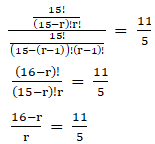5(16 – r) = 11r

80 – 5r = 11r

80 = 11r + 5r

16r = 80

r = 80/16

= 5

∴ The value of r is 5.

10. If n + 2C8n – 2P4 = 57: 16, find n.

Solution:

Given:

n + 2C8n – 2P4 = 57: 16

n + 2C8n – 2P4 = 57 / 16

Let us use the formula,

nCr = n!/r!(n – r)!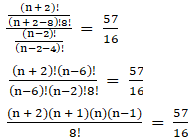[(n+2)! (n-6)!] / [(n-6)! (n-2)! 8!] = 57/16

(n+2) (n+1) (n) (n-1) / 8! = 57/16

(n+2) (n+1) (n) (n-1) = (57×8!) / 16

(n+2) (n+1) (n) (n-1) = [19×3 × 8×7×6×5×4×3×2×1]/16

(n + 2) (n + 1) (n) (n – 1) = 21 × 20 × 19 × 18

Equating the corresponding terms on both sides we get,

n = 19

∴ The value of n is 19.